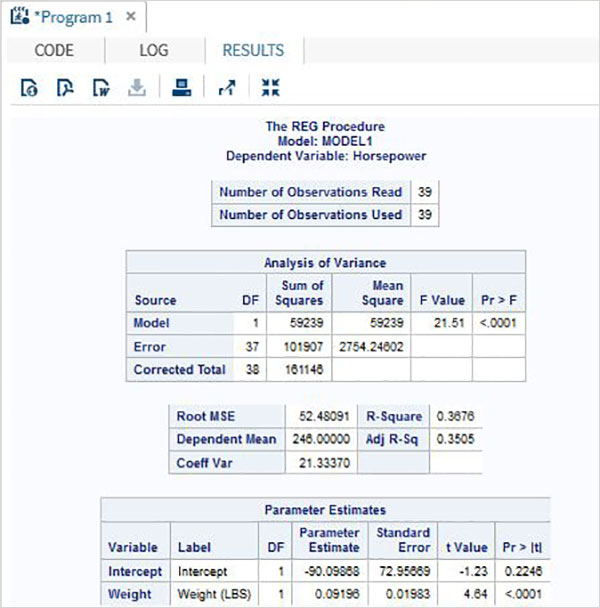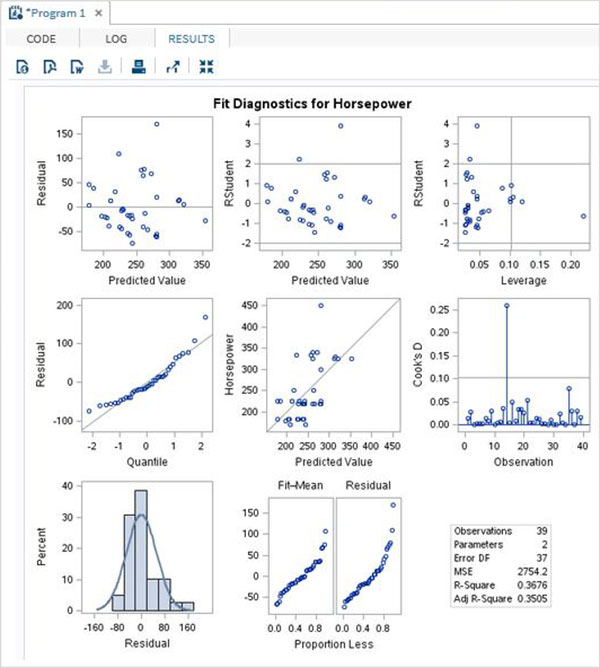# SAS - Linear Regression

Linear Regression is used to identify the relationship between a dependent variable and one or more independent variables. A model of the relationship is proposed, and estimates of the parameter values are used to develop an estimated regression equation.

Various tests are then used to determine if the model is satisfactory. If it is then, the estimated regression equation can be used to predict the value of the dependent variable given values for the independent variables. In SAS the procedure PROC REG is used to find the linear regression model between two variables.

### Syntax

The basic syntax for applying PROC REG in SAS is −

```PROC REG DATA = dataset;
MODEL variable_1 = variable_2;
```

Following is the description of the parameters used −

• Dataset is the name of the dataset.

• variable_1 and variable_2 are the variable names of the dataset used in finding the correlation.

### Example

The below example shows the process to find the correlation between the two variables horsepower and weight of a car by using PROC REG. In the result we see the intercept values which can be used to form the regression equation.

```PROC SQL;
create table CARS1 as
SELECT invoice, horsepower, length, weight
FROM
SASHELP.CARS
WHERE make in ('Audi','BMW')
;
RUN;
proc reg data = cars1;
model horsepower = weight ;
run;
```

When the above code is executed, we get the following result −The above code also gives the graphical view of various estimates of the model as shown below. Being an advanced SAS procedure it simply does not stop at giving the intercept values as the output.